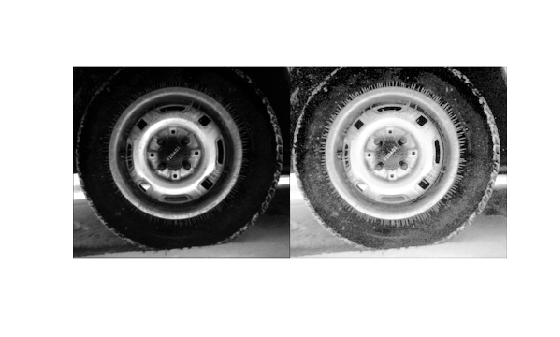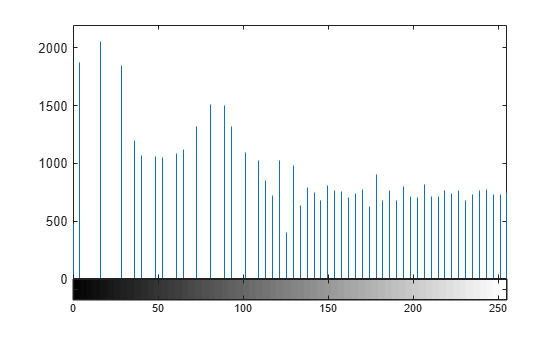histeq

Enhance contrast using histogram equalization

Description

example

J = histeq(I) transforms the grayscale image I so that the histogram of the output grayscale image J has 64 bins and is approximately flat.

J = histeq(I,n) transforms the grayscale image I so that the histogram of the output grayscale image J with n bins is approximately flat. The histogram of J is flatter when n is much smaller than the number of discrete levels in I.

J = histeq(I,hgram) transforms the grayscale image I so that the histogram of the output grayscale image J with length(hgram) bins approximately matches the target histogram hgram.

newmap = histeq(X,map) transforms the values in the colormap so that the histogram of the gray component of the indexed image X is approximately flat. The transformed colormap is newmap.

newmap = histeq(X,map,hgram) transforms the colormap associated with the indexed image X so that the histogram of the gray component of the indexed image (X,newmap) approximately matches the target histogram hgram. The histeq function returns the transformed colormap in newmap. length(hgram) must be the same as size(map,1).

[___,T] = histeq(___) also returns the transformation T that maps the gray component of the input grayscale image or colormap to the gray component of the output grayscale image or colormap.

Examples

collapse all

Read an image into the workspace.

Enhance the contrast of an intensity image using histogram equalization.

J = histeq(I);

Display the original image and the adjusted image.

imshowpair(I,J,'montage')
axis offDisplay a histogram of the original image.

figure
imhist(I,64)Display a histogram of the processed image.

figure
imhist(J,64)Perform histogram equalization.

enhanced = histeq(mristack);

Display the first slice of data for the original image and the contrast-enhanced image.

figure
subplot(1,2,1)
imshow(mristack(:,:,1))
title('Slice of Original Image')
subplot(1,2,2)
imshow(enhanced(:,:,1))
title('Slice of Enhanced Image')Input Arguments

collapse all

Grayscale image, specified as a numeric array of any dimension.

Data Types: single | double | int16 | uint8 | uint16

Target histogram, specified as a numeric vector. hgram has equally spaced bins with intensity values in the appropriate range:

• [0, 1] for images of class double or single

• [0, 255] for images of class uint8

• [0, 65535] for images of class uint16

• [-32768, 32767] for images of class int16

histeq automatically scales hgram so that sum(hgram)=numel(I). The histogram of J better matches hgram when length(hgram) is much smaller than the number of discrete levels in I.

Data Types: single | double

Number of discrete gray levels, specified as a positive integer.

Data Types: single | double

Indexed image, specified as a numeric array of any dimension. The values in X are an index into the colormap map.

Data Types: single | double | uint8 | uint16

Colormap associated with indexed image X, specified as a c-by-3 numeric matrix with values in the range [0, 1]. Each row is a three-element RGB triplet that specifies the red, green, and blue components of a single color of the colormap.

Data Types: double

Output Arguments

collapse all

Transformed grayscale image, returned as a numeric array of the same size and class as the input image I.

Grayscale transformation, returned as a numeric vector. The transformation T maps gray levels in the image I to gray levels in J.

Data Types: double

Transformed colormap, specified as an n-by-3 numeric matrix with values in the range [0, 1]. Each row is a three-element RGB triplet that specifies the red, green, and blue components of a single color of the colormap.

Data Types: double

Algorithms

When you supply a desired histogram hgram, histeq chooses the grayscale transformation T to minimize

$|{c}_{1}\left(T\left(k\right)\right)-{c}_{0}\left(k\right)|,$

c0 is the cumulative histogram of the input image I, and c1 is the cumulative sum of hgram for all intensities k. This minimization is subject to these constraints:

• T must be monotonic

• c1(T(a)) cannot overshoot c0(a) by more than half the distance between the histogram counts at a

histeq uses the transformation b = T(a) to map the gray levels in X (or the colormap) to their new values.

If you do not specify hgram, then histeq creates a flat hgram,

hgram = ones(1,n)*prod(size(A))/n;

and then applies the previous algorithm.